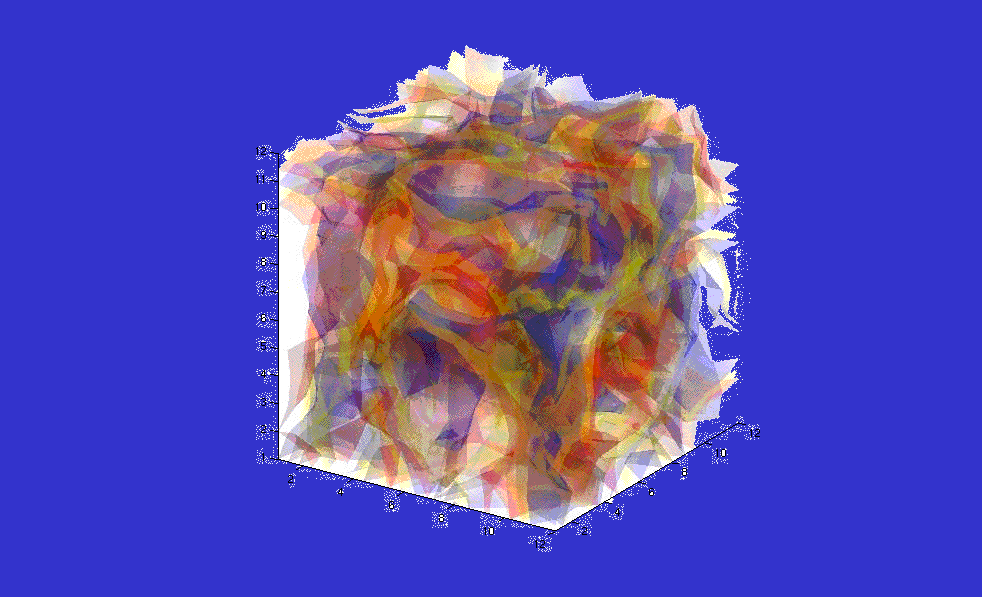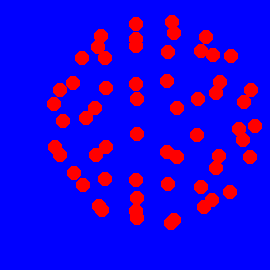Welcome to Matt Kalinski'sHomepageMatt Kalinski's homepage

mkal@mail.pas.rochester.edu
Homepage of world's smallest electric motor (quantum motor) and Quantum Electrical Engineering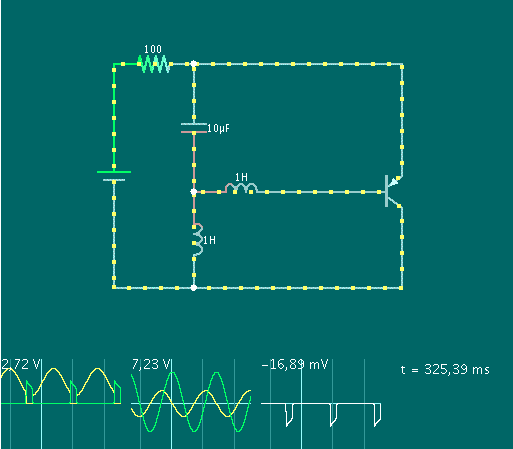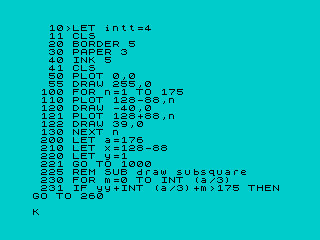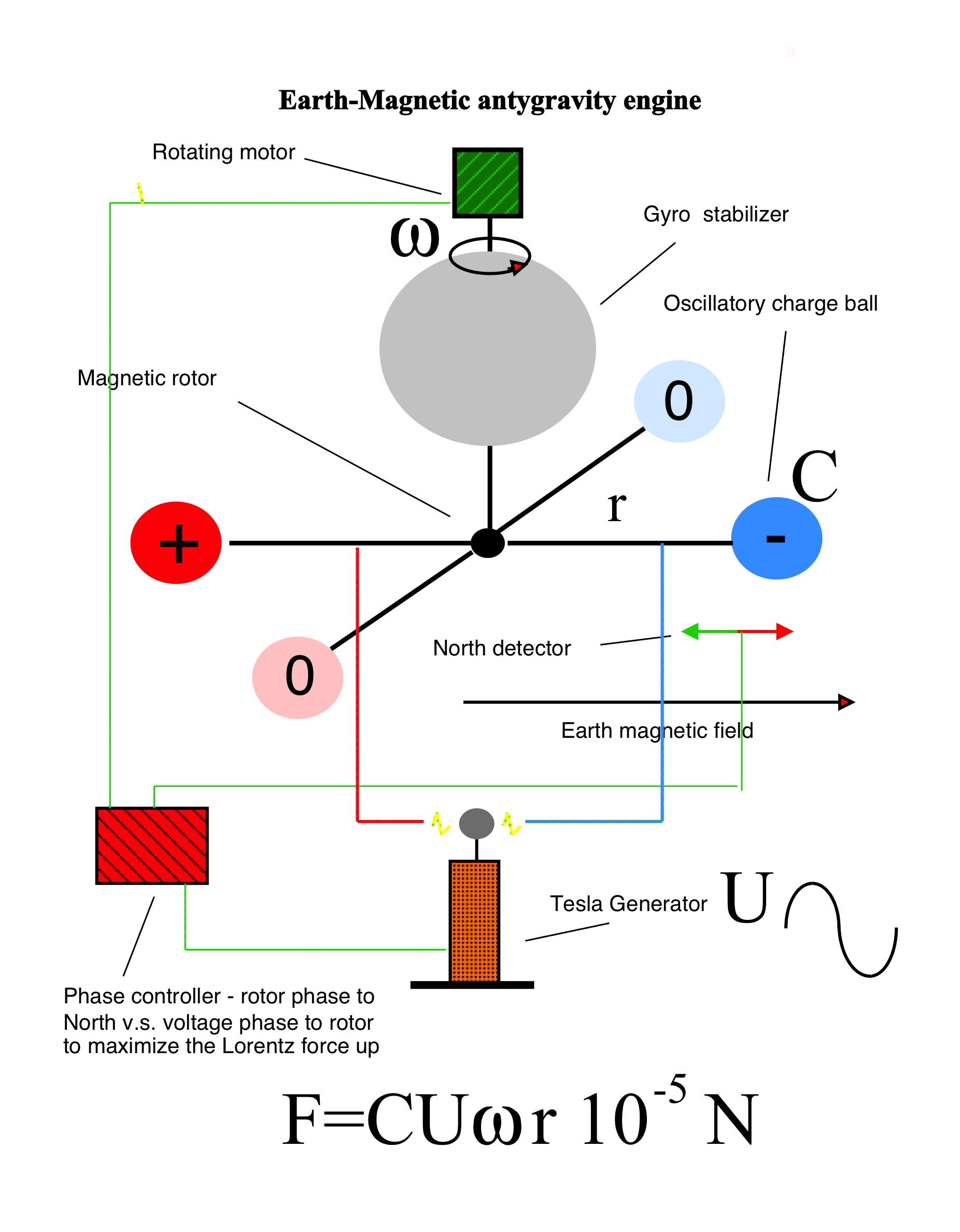In my PhD thesis I designed the smallest electric motor one can build

While the Trojan electron does not stop and scatter and eternally moves with a constant speed the Drude time time is infinite and the Trojan atom is superconducting
While the electron charge as small as 10^-19 C moves around the nucleus with the giant optical frequency 10^14 Hz the persistent current in a single Hydrogen atom is macroscopic 10^-5 A =0.01 mA and the Avogadro number of such atoms 10^24 holds the giant Meissner current of 10^19 A !!!
In the Earth magnetic field of 10^-4 Tesla the magnetic force extorded on such current if in a conductor with only 1 Angstrom lenght is 10^-4 x 10^-10 x 10^19 =10^5 Newtons or 10 tons of force and so in the magnetic field gradiend on a planet with the Earth value per this lenght or simply in the electromagnetic wave timed to the electron loop motion with the same magnetic field amplitude.
Photons seem to be a spin-1 particles with the Hamiltonian being the projection of their spin and their momentum H = p S. Therefore while hitting the objects inelastically they should transfer their internal angular momentum and cause the rotation in analogy to Einstein - de Haas effect . Rigid black body disk will start to rotate under the influence of the Circularly Polarized light (S_z= +-1). The smaller and the lighter the object the better and the certain preexcited single electrons in atoms appear to be the best candidates. My research interests emerge from my PhD discovery at University of Rochester of

strongly localized Starkstates for Zeeman Hamiltonian

so called Trojan states, Landau states for electrodynamics with complex magnetic fields and mass tensor particles.
My PhD: The Hamiltonian of the hydrogen atom in the CP field is H=p^2/2-1/r- e x - w Lz. When w=E2-E1 the matrix of it in the basis of two first Hydrogen eigenstates of the type n=1, l=0, n=0 and n=2, l=1, m=1 (only those two states are degenerate for the exact resonance in the Bloch-Rabi rotating frame for the perturbation theory because of the Lz term) is H= {{E1, e d},{e d, E1}} with the eigenstate |X1>=(|100> + |211>)/2^0.5 and the energy E(1)=E1+d e (Trojan wavepacket)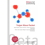and |X2>=(|100> - |211>)/2^0.5 and E(2)=E1-d e (anty-Trojan wavepacket), d=128/243 a0. The first eigenstate is of the type 1 + Exp (i phi) while the other 1 - Exp (i phi) for any fixed r. Therefore the first eigenstate density is like cos(phi) localized around the angle phi=0 while the second is Pi-shifted. The radial localization is due to the overlap between two hydrogen states in r. Very clear banana-like shape of the packet can be obtained in two-level approximation when one elevates the excited n=2 component of the state by small detuning from the energy difference so the relative radial contributions from both states are equal at overlap between a0 and 4a0. One may therefore notice that the different energies of Trojan and anty-Trojan wave packet is also the Autler-Townes splitting. For the larger and larger n (w=E_(n+1)-E_n, l=m=n-1) wavepackets sharply focus relatively to the atom size as the 1/n effect.
In numerical experiments and without any analytical theory the hydrogen circular state simply behavies like it wants to touch you. When you tease it with the resonant CP field and the force against you it sharply polarizes into the opposite direction towards you.
While considered within the harmonic appoximation Trojan wavepacket ionization is equivalent to the Dicke-Hepp-Lieb superradiant phase transition when the true collective field-matter electromagnetic vacuum collapses and "Mexican hat" stabilizes in displaced state by the nonlinearity under the electromagnetic interaction when the density of oscillator matter exeeds critical
While considered within the Mathieu theory and spanned by the circular eigenstates of Hydrogen it is a direct example of Poincare reccurence theorem in a simple system when the full exact quantum revival and Poincare reccurence time of zero-field evolution after the creation became one for the interval being devisible by the all squares of the quantum numbers in the expansion at once. Even for quantum numbers as low as 60 it becames hyperastronomical and therefore the Poincare time (10 to power 70 years).

When the relative dielectric susceptibility of matter X is -1 its relative dielectric constant epsilon = 1 + X is 0 (i.e. less than for the vacuum 1) and according to the screened Coulomb law the infinitely small external field from the point charge will cause the infinite Coulomb 1/epilon r^2 force and will induce the infinite internal electric field E=D/epsilon and the infinite opposite polarization causing it. Because of the NEGATIVE effective mass of the electron (anti-electricity in analogy to anti-gravity) this is the case of the Trojan matter. In practice because of the nonlinearity of response the polarization due to the infinitesimal field will saturate to finite. When considering the collective effects Trojan wave packets from their dipoles can generate together the internal electric field which consistently supports them so the external field does not need to be present. While the atomic dynamical response (Nonlinear unisotropic parallel resonant response theory of Hydrogen Circular States below ionization) and the polarizability of the Trojan state at the driving frequency is anomalous and negative (the dipole moment of the atom like a growing plant towards the light polarizes Trojan dipoles in the direction opposite to the electrostatic force from the capacitor plates charge surface density acting on the negative charge which normally orders dipoles to grow the capacitance) i.e. opposite to that of the normal dielectric at static response and so their electric susceptibility X_0(omega_0) <0 and they are both nonlinear and strongly depend on the external electric field under the resonant distance conditions between Trojan atoms the gas can self-lock on nonlinearity without any external field (While matching the outer empty space no normal field D=0 boundary condition for a Trojan electret stlab instantenously D = E - 4 Pi P(E) = 0 => E.ne.0, P(E).ne.0 i.e. the polarization is fully creating the internal electric field to cause it, above the critical Trojan dipole density and P(E) is fast-increasing and strongly nonlinear with "transistor Ic vs. Vc" saturation) and the native state of interacting Trojan gas is dynamically ferroelectric (superconducting with ethernal intra-atomic Meissner-like sigle-electron persistent currents). The two simplest three dimensional lattices supporting the native Trojan ferroelectric (and not anty-ferroelectric) order (all packets move in phase) on the microscopic Clausius-Mossotti cluster level (locked only on local field) are primitive tetragonal lattice (direction stretched (here compressed) simple cubic lattice) and parallel hexagonal lattice - Trojan hydrogen atoms are on honeycomb Graphene-like two-dimensional lattices parallel to each other and closer than the critical distance related to the intra-plane distances matching the atom positions projections perpendicularly and for both with packets move in planes. When the finite temperature is considered the temperature averaged quantum states being the thermal superposition of the circular Trojan manifold states will under the Einstein B coefficient (de)-excite towards low dipole moment rotational states and therefore new kind of the superradiant first order phase transition occures (nuclear-like shape transition) from non-ferroelectric (circular states with zero polarization) to ferroelectric (Trojan states) state when the temperature changes and the dipoles become thermally insufficient. Because once localized by the field the dipole moment of the Trojan wave packet saturates to its semiclassical value r_0 e (where r_0 is the Trojan Wavepacket orbit radius) and further almost does not depend on the field electric i.e. self-consistently E = E_sc/r_0^2 = - P = N/V e r_0 = 1/a^3 e r_0 (where a is the distance between the nearest Trojan atoms and Esc<0.11, a^3 is per-dipole volume) the value of the critical parameter N/V e^2/m w^2 around 1 (omega is the main oscillator frequency i.e. the Kepler frequency of the packet) remains the same that for the normal ground-state Lieb superradiance superradiance which is also the same order (1/3 of that) when the susceptibility becomes infinite on singularity due to the positive polarizaion feedback from the spherical dielectric hollow within the simple Clausius-Mossotti theory N/V e^2 / 3 m w^2 = 1 when the polarization centers are simple harmonic oscillators.Classical Trojan Wave Packet Simulation using 1982 3.5MHz 48kB ZX Spectrum:
The Sinclair Basic code:No CP field (spreading):CP field ee=0.04 (non-spreading):Simple man theory of Trojan wavepacket
Trojan wavepacket viewer
I therefore engineered the smallest electric motor one can build with dynamic fields design (with a single atom) !!!
In normal electric motor (DC) magnetic field changes around rotor adjusting the torque to maximum for given phase (using the switch called commutator )
In inductive or dynamic magnetic field electric motor (AC) the magnetic field of the stator rotates around rotor with steady magnet (no commutator is nessesary)
In my motor the rotor is a displaced electric charge (dipole) not a magnet (it is actually the electron on circular Kepler orbit) and the electric field is rotating instead of magnetic (the stator is the nucleus) - you can also consider it as simplest example of electrostatic propulsion on atomic scale

Like in classical macroscopic electric motor the bearing is not frictionless but the friction is with the electromagnetic vacuum modes - is radiative
Quantum motor while it works:Mechanical model - electrostatic Coulomb force extords the torque instead of magnetic forces - first designed by Physicists Benjamin Franklin and Johann Christian Poggendorf
Trojan motor as circulating current has the magnetic moment and is subjected to forces in the magnetic field gradients amd therefore is a microscopic Flying Gyro experincing the magnetic antygravity
You can build a hybrid at home and power it with your TV screen !!! or Van de Graaff generator (DC version)
I spent 6 hours tunning mine so be patient ... - focuse on bearing smootness (Marklin car bearing seems the best if you have time beyond ball pen bearing) and the sharpness of commutator needles (just needles)
(Or you can call it photon wind power plant if you like)
The tricky point is that with the quantum matter it is not so simple - the rotor is really liquid and like a droplet of water exists only within a fine parameter range (sizes of the droplet versus the electric field strength). (see the theory above).
The macroscopic applicable electrostatic motor whould have something between the hard drive technology, high voltage capacitor technology and the display technology - the volumetric multi-cylindrical capacitor arrays in almost superfluid dielectric with thousands of electronically switched sub-sectors and hundreds of sub-surfaces should be the best.
Old US patents of electrostatic motors
and now more complicated engine - Langumuir states are physical in crossed fields !!! APS
Making Trojan wavepacket (or quantum motors) costs 500000 dollars but it generates "visible" Hawking radiation in muonic Hydrogen
Since is accelerated with 10^25 g there
My free "ticking" symplectic solver for atomic clusters and astrophysics (forms Kepler "Cooper" pairs each time step) click the grey movie to downoad, there is no Makefiles but you can alter it

This how the atoms get the kinetic energy during Coulomb explosion so the cold fussion occurs in the Deuterium clusters micro-fog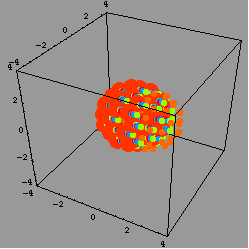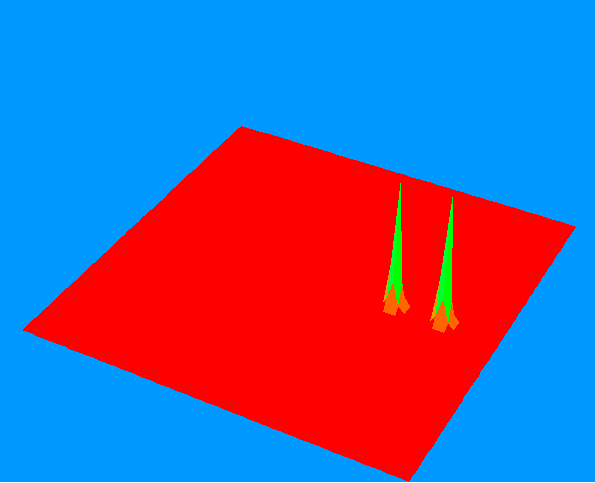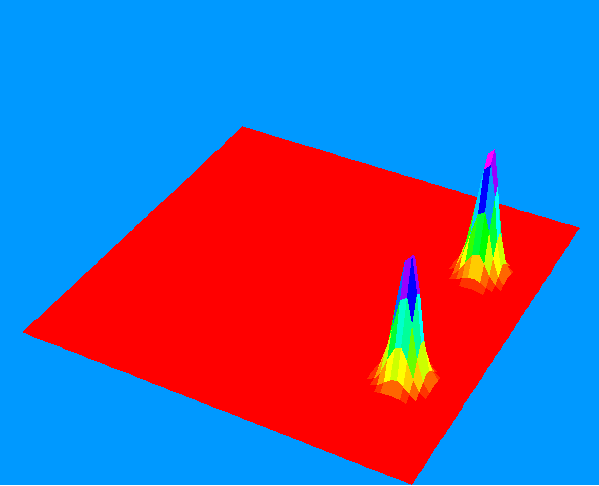Two-electron pairs orbiting in strong magetic field (or electron-positron pairs in CP field). As the wave functions do not overlap the recombination process can be frozen indefinitely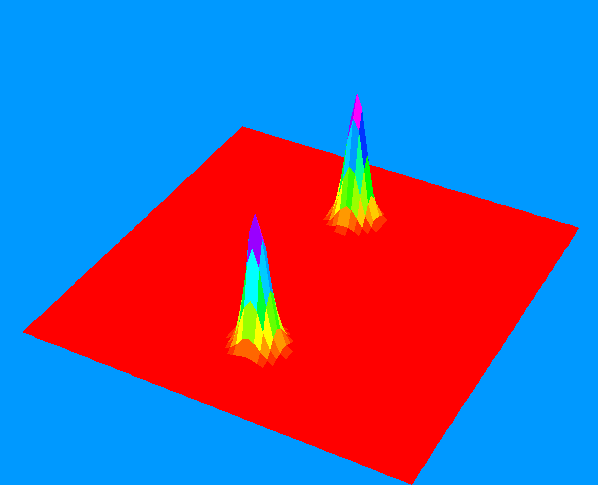Other small but not yet quantum motors
Enzyme powered rotating γ subunit
Metal droplet motors
Single molecule motor with hydrogen-carbon chain rotor and sulfur-copper bearing powered by tunneling microscope
Molecular B13+ infrared ionic conductivity bicycle motor with chain powered by the CP field with 13:1 derailleur in asymmetric Wankel configuration
Carbon nanotube bearing nanomotor powered by exchage of surface electrons angular momentum
Other quantum but not electric motors
Brownian motors - climbing with fluctuations
Carbon nanotube steam locomotive-like nanomotor powered by temperature gradient

Like the model trains initailly localized wave packets will move on periodic trajectories like on a track spreading fast on unstable trajectories and not dispersing on locally stable trajectories. While all trajectories are points in a certain instatenous rotating frame and the WKB wave functions spike singularly around the turning points of zero velocity there must be localized eignstates there around to phase interfere. The locally rotating frame augmented to the trajectory tangent circle may be used to study adiabatic stability. I am looking for various iteresting, for example 8-shaped trajectories which can support on-average nonspreading Trojan-like wave packets. Triangle, square and general polygon trajectories are possible in atoms in combination magnetic and electromagnetic fields for one and more electrons.

Interested in selected exotic eigenstates, I am currently working on Monte Carlo genetic algorithm implementation of fixed node method. The nodal constrains are tossed randomly and than the genetic operations are performed on best nodal populations to produce better energy estimate of selected excited states and so on.
You can also use it with tossed-node imaginary-time split operator method
Studying the time dependent free dynamics of the Trojan wave packets after the sudden field turn-off I have recently discovered that the full quantum revival occurs in any quantum system with the rational energies and therefore asymptotically in near-ifininity in any quantum system. Thus it is equivalent to the Poincare revival. While the normal revival considerations expand the spectra up to be harmonic or quadratic as for the quantum well for the Trojan wave packet the validity of expansion is equivalent to the expansion truncation. Depending on the number of states involved in the time evolution expansion the exact revival time of the Trojan wave packet becomes the Poincare when it is as long as non-physical on the Earth laboratory time scale.

 American Physical Society Apple Cray Research Optical Society of America Silicon Graphics Wolfram Research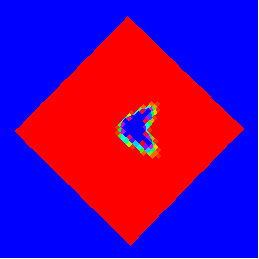My hot topics for today:
Quantum theory of dielectric constant of organic materials
Inertial confinement of solid hydrogen
Theory of dielectric constant of Bose condensate
Superradiance of Rydberg gases in quantum cavities
Car-Parinello simulations of egzotermic reactions
Cellular automata by quantum dots arrays
Physics of Magnetars
Fussion in atomic clusters in strong fields
Quantum monodromy in helium in crossed fields
Spontaneous light amplification through superradiant emission of radiation (SLASER)
Non-fixed node Monte Carlo with genetic algorithm
Bose-Hubbart theory of color Bose and mixed gases
Nonlinear acoustic subsceptibilities of Bose Gases
Beta electron beams from on-wave acceleration
Detection of heavy mases with mesoscopic SQUIDs
Unruh-Davies effect from quantum entanglement
Ionization Kondo effect in Rydberg gases
Mesoscopic superconductivity
Split-operator methods for spinors and wave function of photon in dielectric media
Contraction-of-propagator methods for protein folding
Quantum computing with quantum dots
Trojan wavepackets with Wannier excitons
Spontaneous order in systems with negative mass
Superradiance with mouonic hydrogen with cyclotronic motion
Spacial control of dielectric constant
Optical phase solvers of Schrodinger equations
Quantum entropy ordering with Trojan wavepackets
Ticking of quantum states
Quantum entropy fluctuations in systems with anomalous spectra
Chaotic oscillations of quantum states in rotating systems
Channeling in multi-center scattering
Model Born-Infeld theories with electromagnetic vacuum collapse
Bohm Hydrodynamical Quantum Mechanics and real time Diffussion Monte Carlo
Dicke superradiant phase transition as supersolid formation in Bose-Einstein condensate with oscillatory interactions: uniform(normal) versus oscillatory (superradiant) phase
Cactoo fractals
Configuration existence theorem for N electrons in magnetic and circularly polarized fields: The maximum number of configurations may be the product of all differential foldings (maximum number of times the ZVS gradient manifold is cut by a straight line to the separate points): There may be at least 2^180=1532495540865888858358347027150309183618739122183602176 maximum number of configurations of electrons corresponding to carbon C60 (N=60) assuming the lowest nontrivial folding 2 (parabola-like). The same applies to the molecules consisting of atoms if the binding forces can be assumed to be conservative and have the potentials and their all components have notrivial folding at least 2: There may be in principle 2^(3 N) distinct molecules consisting of N atoms i.e. for example 2^180 allotropes of the C60 buckyball molecules (like 3 x 20, 5 x 12 or 6 x 10 sheet of Graphene or 60-atoms short Carbon nanotube etc.). To see this theorem working in 3D one may consider a rose and two planes as some differential ZVS gradient manifolds. The rose has clearly high folding and then when cut again perpendicularly is cuts to a lot of points. In 4 dimensions the line can cut the perpendicular or non-containing 3D space at 1 point (the system of 4 linear equations - 3D space equation in 4D + the line equation has only one unique solution) and therefore the 4 dimensional ellipsoid a x^2 + b y^2 + c z^2 + d t^2 = e in 2 points so there is maximum 2^4 = 16 solutions of the system of nonlinear equations consisting of 4 ellipsoid equations embadded 4 dimensional space or otherwise analytically when such system of equations is linear for x^2, y^2, z^2, t^2 it has only one nontrivial solution for them and if they are all positive it has 2*2*2*2=16 solutions for (x,y,z,t) because of the double sign of the square roots of the former.
Concentration of primes: 469!-1 was once found prime and 469! - 1 -4362, 469! -1 -4902, 469! - 1 -5406, 469! -1 -5652, 469!-1-8580, 469! -1 + 1130, 469! - 1 + 2084, 469! - 1 + 2592, 469! - 1 + 6662, 469! -1 + 6998 are all prime !
11^1008 + 998672782 was once found prime and so IntegerPart[2(11^1008 + 9986727 82)^2 - 1)^0.5] + 3695 is.
Exact revival of the wave function of mathematical Hydrogen: The arbitrary wave function (not only Gaussian wavepacket and approximately) will recover exactly for the system with the energies En=-R/n^2 after the time T=2 Pi hbar Lm /R where Lm is the lowest common multiple of squares of all quantum numbers involved in the wave function. When first 120 states are involved this is about 10^79 years !!!
Generation of Langmuir Trojan states in Helium: First the Trojan wavepacket is generated from one electron leaving He+ ion core, the plane of motion is adiabatically shifted by the static Stark field turn on parallel to magnetic field and then the second Trojan wavepacket from Helium He+ ion is generated but to much smaller off resonant orbit not much to influence the first one. Later "two color" CP field is adiabatically adjusted to one frequency while turning off the asymmetric Stark and turning on the magnetic field.
Barnett-Einsten-de Hass (rotationally induced) quantum Hall effect: When Trojan wavepackets are build from Wannier excitons and confined in quasi two dimensions the rotation of the sample will induce alternating Hall voltage along the thickness due to normal ridid body rotator response in this direction. When the number of Trojan excitons is quantized the Hall voltage will.
Detection of gravitoelectromagnetic F= v*dm/dt*(Gm^2/r)/(2 Pi m c^2) Lorentz force with gravitational Aharonov-Bohm oscillations in Trojan wavepackets. For Trojan atom placed on the fast satellite orbiting Earth within about 1 hour in the Trojan Michelson-Morley experiment the possible gravitoelectromagnetic flux due to the Earth existence will shift the electron interference pattern for n=600 by one full fringe during 1 year !!!
Trojan Hydrogen as Josephson Junction through Quantum Phase Model (QPM)
"Compressed sping" phase transition in Trojan elliptical harmonic two-electronquantum dots
Coherent tunneling between two Trojan atoms in two ultrashort delta laser pulses
Trojan wave packets from electron gas in the Coulomb field of higly charged ions in ultrastrong CP laser field
Uncertainty relations for excited Trojan wave packets
Exact revival of the wave function of arbitrary quantum system with rational energies. The quantum wave function will recover exactly for the system with the energies En=C Nn/Mn after the time T=2 Pi hbar Lm /(C Ld) where Lm is the lowest common multiple of all denominators in fractions in energies involved in the wave function and Ld the greatest common divisor of the numerators (most probably 1 for long sequences with large primes). Since the set of the rational numbers is so called dense in the set of real numbers (for any irrational number there is the rational one arbitrary close to it) each quantum system revives exacly on theoretical computer with truncated accurancy since any number there is integer/10^n. Example 1) Jaynes-Cummings Model: Consider the energies of Jaynes-Cummings model En=(n-1/2)omega +- (1/2)[(omega-Omega)^2 + 4 g^2 n]^(1/2). To make energies rational (integer here) let us assume the state is localized in n and spanned around the principal quantum number n0. The energy Taylor expansion around the leading state n0 is En= (n-1/2)omega + Const + g^2/[(omega-Omega)^2 + 4 g^2 n0]^(1/2)(n-n0). While the coefficient multiplying (n-n0) is small we can assume omega is also the integer multiple of it. While only even or odd ns are involved (the eigenstates mix the consecutive photon numbers) we get the revival time (to revive the state up to the phase factor) T= Pi*[(omega-Omega)^2 + 4 g^2 n0]^(1/2)/g^2 Example 2) Hydrogen atom for shorter times: Consider energies of hydrogen atom En=-1/2n^2. Let us assume that the quantum state is localized around the principal quantum number n0 (Gaussian-like in n around n0) and expand En=-1/2n0^2 + 1/n0^3*(n-n0) -3/2n0^4*(n-n0)^2 to make it as varable integer multiplying the common rational. Since the coefficient multiplying the quadratic term is small to the Kepler frequency 1/n0^3, the Kepler frequency 1/n0^3 can be assumed the integer multiple of it. Without the loss of generality we can assume expansion in n-n0 as even. Therefore the revival time is 2*Pi*n0^4/3.
Superrevivals in Jaynes-Cummings model: Mapping the revival dynamics of the Trojan wave packets after the sudden field turn-off onto the Jaynes-Cummings model the revivals in J-C models are full rotations of the spreading Trojan wave packet while the full revivals of Trojan wavepacket are superrevivals in Jaynes-Cummings model after the time T= Pi*[(omega-Omega)^2 + 4 g^2 n0]^(3/2)/g^4 when the normal revivals will to reshape back to the original decay and around. The superrevival time is about n0/g^2 longer then the revival time !
Exact superfast revivals of special quantum states in Jaynes-Cummings model on resonance: Let on resonance En =(n-1/2)omega + Const +- g n^(1/2) and omega is integer multiple of g then any quantum state such that n = kn^2 revives exactly after the time Trev= 2*Pi/g when Trev* g* 2*Pi*n^(1/2) = 2*Pi*kn . Specifically the incomplete coherent states with the number state expansion holes and n components quantum numbers being the square will revive fast and exactly. If kn are localized around large kn0 then kn^2 \approx kn0^2 + 2*k0*(kn-kn0) and n are approximately even or odd with the constant gap.
Looking for arbitrary primes using the exact revival of arbitrary quantum system with rational energies: If En= C Nn/Mm (Nn=1, Mn=n^2 for Hydrogen) has Mn as the power of prime then the superposition of N such consecutive levels has the autocorrelation function always below 1 during the time 0 and the exact revival time 2 Pi hbar Mp1*Mp2...MpN/C (lowest common multiple of primes is their product) so if all first N of primes (really those up to MpN+1/2) is known the next bigger N-th+1 can be found from no reaching 1 condition (or is MpN+1 a prime ?).
Phase shifted exact revivals of the wave function. Let En=C/n^2 and the wave fuction is the superposition of such n that n=10^kn. Let knmax is the maksimum of kn in the wave function expansion. Lm=10^2 knmax and the exact full revival occurs after time T=2 Pi Lm/C but since for the each integer 10^2 kmax/3, 10^2 kmax/3=3333...3 + 1/3 the phase shifted exact revival such that Psi(T)=Exp(i 2 Pi/3)Psi(0) happens already after the shorter time T=2 Pi Lm /3/C.
Arbitrary state preparation with the exact revival of the wave function: Providing that the amplitudes of the wave function are first prepared the arbitrary phases can be reached only by waiting for the sufficient time of the quantum evolution as the time of the full revival with the energies renormalized by the inverses of the rational phases up to full multiples of 2 Pi or the wave function phases sweep the whole asymptotically infinitely dimensional phase hypercube modulo 2 Pi for the arbitrarily long time.
New method of Trojan Wavepacket generation: 1) Arbitrary Gaussian distribution of the circular states is generated around the resonant state. 2) Free no-field evolution is allowed till the Trojan wave packet is self-formed. 3) The parameter matching CP field is turned on instantaneously in phase.
Quantum gates with two Trojan atoms coupled by dipole-dipole interaction with Trojan and anty-Trojan states as 0 or 1.
Dynamic ferroelectricity and antyferroelectricity in the system of interacting Trojan atoms.
Dirichlet problem for the d'Alembert wave equation with one periodically oscillating wall: Let u(x,t) is the solution of the wave equation and u(0,t)=0 on one rigit wall at x=0 and further u(q(t),t)=0 with q(t)=a+b*sin(w*t). Let f(x) = x + 2*a + 2*sin(w*(x+a)). Then u(x,t)=g(f(f(f(...(t-x))))-g(f(f(f...(t+x)))) with the finite multiple Feigenbaum f composition is slow on convergence to the fixed point or differs a little on the next interation and is the approximate solution when g(x+2*a) = g(x) i.e. g is periodic with 2*a or exact for u(h(t),t)=0 with h(t) periodic and close to q(t).
Quantum scars in infinite stadium potential well as quantum carpets for one-dimensional Klein-Gordon equation with the imaginary time and time-dependent semi-circle boundaries u(q(t),t)=0 with q(t)=+-[a+b*\$in(t/b)], \$in(t)=[1-(1-t)^2]^0.5. The scar energy is the square of the mass energy of the pseudo-relativistic particle at rest. The scar reflection from the parallel stadium edges is the Klein paradox with the particle probality coming out from everywhere in the whole volume (or the one-dimensional space) due to the imaginary time or propagation velocity.
Kondo temperature suppression of ionization of Trojan Hydrogen as Superradiant Phase Transition.
Trojan wave packet as the superradiant electron state of the Jaynes-Cummings model after Holstein-Primakoff transformation for two levels
Quantum Hall effect and annihilation suppression in electron-positron gas
Cold fussion of magnetically stabilized two-nuclei Trojan states of Deuterium
Slaloming of Trojan wave packet of Deuterium nucleus in Palladium crystal lattice
Ionization probability dependent interractive intelligent grid renormalization method for strong field ionization problems # => []. Each time step the probability to find an electron is calculated in the outer near-boundary spatial region. When the critical is reached the size of the spacial grid step is doubled and the wave function is rewritten from the previous grid each second grid point being again far from boundaries.
Trojan stability paradigm in harmonic two-electron quantum dots in electric and magnetic fields emulating rotation.
Soft chaos induced shape life of Trojan wave packets due to Henon-Heiles like corrections and randomness of "irrelevant" Stark-Zeeman energy levels.
Dynamic ferrolectricity of Trojan hydrogen atoms on 2D honeycomb lattice (Elok > 0).
Nonspreading wave packets of near-luminal electrons in free space. According the relativistic energy-momentum relation E^2= p^2 c^2 + m0^2 c^4 the dispersion relations E(k) for free electron and positron form the avoided crossing with the energy gap being twice the rest mass energy of the electron m0 c^2 or the energy of the pair creation. As the result they become linear and photon-like in utra-reltivistic limit k -> Infinity and Trojan-like wavepackets exist in free space moving with almost the speed of light. They can be generated from Trojan wave packets (focuser) by ultra-strong phased electric field delta kick applied to Trojan electron (accelerator).
Effective Hartree-Einstein equation for the relativistic electrons: p^2/(grad S(x)^2/4 c^2 + 4 m0^2)^(1/2) + V(xt) + m0 c^2 = i d/dt, Psi(x) = |Psi(x)|exp[iS(x)]
Rotational "Third force" on Trojan wave packets: In Electrodynamics there is other than the Coulomb Force and the Lorentz Force force acting on particle without the electric charge but with the dipole magnetic moment and with the spin (e.g. on neutrons) in the crossed combination of the electric and magnetic fields. Because the free evolving spin with the magnetic moment undergoes the precession according to Bloch equations i.e. the end of its vector axis moves around the circle and its tilt angle with respect to the magnetic field vector is constant it also generates the magnetic field which is time dependent. This field is generating the magnetic flux which is variable in time and according to the Faraday's induction law and the Special Theory of the Relativity it is seen in the laboratory frame as the combination of two fields, the magnetic and the electric one. Now if such spin is placed between the plates of the charged capacitor the variable in time magnetic flux from the dipole motion will induce forces acting on rigidly mounted electric charges. According to the III Newton's principle of dynamics about the reciprocity of force action this force also acts backwards with the same strength on the magnetic dipole. In the extreme condition of the precession angle of 90 degrees this force is therefore in the direction perpendicular to the electric field and parallel to the magnetic field. Such capacitor however is equivalent to the constant electric field generated by its charged plates. In general case of the electric and magnetic field orientation the third force can be expressed as: FIII=1/mc E x (m x B). The similar effect will be for Trojan wave packet when the electron spin is precessing under Eistein-de Haas-Barnett interaction in the rotating frame.
Intrinsic Trojan-like i C x y entanglement in quantum systems and spin 1-space coordinateentanglement in the wave function of photon
Violation of angular momentum Bell inequalities for two-atom entangled Trojan cat states
Electron free fall in the nuclear electric field and strong magnetic field: Trojan-like wave packets on Gryzinski-like trajectories in linearly polarized electromagnetic field with variable polarization plane.
Langmuir Trojan-like electron configurations on triangular, square and regular polygon- trajectories in strong magnetic and circularly polarized fields
Direct determination of the vacuum impedance from the existence of Trojan like wavepackets on regular poligon trajectories in the magnetic field
High harmonic generation from Trojan-like electrons in strong magnetic field on polygon trajectories due to near-infinite vertex acceleration
Writing with the electron nondispersing wavepacket orbit in strong time dependent magnetic and electromagnetic fields
Detection of Lense-Thirring effect with Trojan atoms: Above the Earth poles the antygravity experienced by the Trojan atom with the the electron rotating in the Earth spin direction is equall to (2/5) n alpha^2*/(omega_Earth/omega_Bohr) g where omega_Earth is the 2*Pi(3600*24) Hz Earth rotation while omega_Bohr is the first Bohr orbit frequency and while (omega_Earth/omega_Bohr) is measurable it is readily measurable for ultra-Rydberg atoms with n = 1/alpha^2 = 137^2.
Cavendishexperiment for sigle atoms: Single electron cat states in the Hydrogen Rydberg pseudo-molecule to detect electron-proton gravity through gravitational Stark effect on Trojan wave packet
Delta pulse ionization current field effect single atom transistor (FET) using Trojan-anty Trojan dynamics
Direct exact violation of the Second Law of Theromdynamics by the spontaneous lowering of the quantum vom Neumann entropy in the ultra-long field-free time evolution of Trojan wave packets
Internal coordinate exitations of Trojan wave packet as the Hawking radiation
Attosecond Transient Absorption by Trojan Wave Packets
Intensity dependent dielectric constant theory of incoherent Trojan wave packets: Assuming the Gaussian density operator around the central state within the Trojan energy manifold of circular states the energy balance between the C.P. field absorption and the spontaneous emission predicts the existence of the ethernal localized density Trojan wave packets in the decohering thermal reservoir even when the off diagonal elements of the density matrix are zero.
Microwave superconductivity of Trojan electron-positron pairs at finite temperatures
Trojan Positronium formation during electron-positron Bhabha scattering in the laser field: The first step towards the Trojan matter-antymatter production is non-recombinating positronium in the Trojan wave packet state. In the first experiment the cathode positron anode ray tube providing low energetic beams of electrons from cathode and positrons from anode covered by the positrons producing radionuclide are aligned to collide in the center between te cathode and the anode. While the collision point is subjected to the microwave or the laser circularly polarized field finely tuned to the beam velocities and lepton beam densities the Trojan positronium formation should occure to stop the matter and antymatter currents to pass through the collision point and reduce the annihilation and the gamma radiation.
Kronig-Penney (K-P) model of supersolid formation with insulated delta potential: While the singular delta attractive interaction is added to the rectangular potential barriers and both are taken as the interaction potential the Gaussian solitons formed because of the delta attraction while trying to tunnel organize in periodic structures to form supersolid in a weak external harmonic envelope potential: Stationary Gaussian solitons droplets form because of the locally solitonic Gross-Pitaevskii equation, they repel with the K-P potential while are bound by the Bether-Peiers-Weiss potential from neighbours.
Hand writting with Landau states: Because there is a symmetric gauge A = (-B/2 y, B/2 x , 0) in which the potential of the quantum particle in the magnetic field is symmetrically harmonic with the diamagnetic term with the oscillator frequency proportional to the field it is possible to drive the Gaussian Landau state along the arbitrary trajectory using the exernal potentials (time-split the propagator as Landau and the external potential). First the paramagnetic omega_c/2 L_z term may be eliminated by the equaivalent counter-rotation of the coordinate system and the new time-rotated trajectory is the arbitrary for the Ehrenfest inverse dynamics problem for the ordinary potential for the Gausian packet. It is why the extra strong magnetic field is improving the spatial confinement of Trojan Wave Packet by x-reverting the stable rotating saddle point into the potential diamagnetic minimum and making it more orbiting Gaussian Landau state than the Trojan Wave Packet itself. In two dimensions because the quantum and the classical time evolution equation for the Wigner function (i.e. clasically the Liouville phase space distribution) is identical for the harmonic oscillator which as quantum can only have piecewise negative Wigner functions Trojan Wavepacket is stabilized to exist for arbitrary low resonant quantum number by the pendular quantum nonlinearity and Trojan-like wave packets exist without the nuclear hydrogen Coulomb field as simply C.P. field accelerated Gaussian Landau states when one turnes-off the field of the hydrogen nucleus. It is possible to find the time dependent CP field such that arbitrary trajectory including hand written messages can be achieved in time first found by the inverse problem. While the changable width of the Gaussian is pemitted the time dependent magnetic field may be also used in combination. Some kind of trasverse confining potentials like the grid of Coulomb potential exends the method to three dimensions.
Weak excitations of uniform Gross-Pitaevskii condensate with ultra strong self-focusing interaction
Stability of true quantum cubical atom of Oxygen in electromagnetic fields. While the exact static cubical atom with no fields in the vacuum is the ion with only the fractional nuclear charge Z=(1+3*3^0.5+6^0.5/2)/2=2.4676... (end always unstable according to Ernshaw´s theorem the physical integer nuclear charge maybe tuned either by the symmetric screening with the dielectric constant cubic inner zone (precise - not Menger Sierpinski sponge (fractal dimension Log(26)/Log(3)=2.96565... > Log(20)/log(3)=2.72683... ) 1-st interation (by removing (altering the dielectric constatnt in) only the central cube in lower partition)) or by the spherical harmonic quantum dot potential.
Collective"Hydrino" flakes: Storing chemical energy in Honeycomb self-sustained Trojan Atom clusters (Hydren) approximately 13.6 eV per excited Trojan atom (N*13.6 eV per N Trojan Hydrogen atoms)
Trojan wave packets in the quantum cavity as the ethernal, non-collapse and no-revival electron-photon superpositions immune to the spontaneous emission: Jaynes-Cummings-like model for the infinite number of slightly off-resonant quantum levels: Our Mathieu theory can be extended to the full electron-photon system with the quantum electromagnetic field. The states with the fixed deviation of circularity may be multiplied with the Fock states with the fixed number of photons and the augmented polarization in reversed quantum number order providing the proper Jaynes-Cummings ladder energy exchange conditions. The collective pendula in the electron-photon space are immune to spontaneous emission. Collective electron-photon Trojan wave packets with the discrete level of confinement as the new quantum number dependent on the photon-electron ladder offset in such space are fully ethernal and immune to the radiative decay and are the single electron Meissner effect for the photonic superconductivity. While the circular energy states interact with the resonant modes of the cylindrical cavity the harmonization of total Jaynes-Cummings spectrum occures. The field-electron superposition is the collective Schroedinger-Lorentz coherent Brown state for the Hydrogen-cavity with the non-spreading electron density and without the electromagmetic decay as only the phases of the circular states evolve but not the populations. While the dressed pendular states are stationary in the laboratory frame the coherent superpositions of states with the different photon number offset (for example with the coeeficients of the corresponding photon coherent states) with respect to the circular state running quantum number and with energes that now differ exacly by the harmonic hbar omega are nondispersing electron density wave packets. While they are photon-electron collective they are ethernal also with respect to the spontaneous emission. New method of no transient absorption spectroscopy detection of Trojan wave packet is implied with Positronium Trojan atom: Positronium Trojan atoms in presumably Trojan state are injected to quantum cavity with compatibile quantum C.P. field to maintain them in the Trojan states indefinitely. While the cavity has finite Q the Klystron power injection is nessesary. After Klystron power turn off the enhanced gamma emission from the recombination should be observed while the positronium was Trojan.
Ionization lifetimes of Trojan Wave Packets with hypergeometric-coordinate method appplied to normal circular or spherical coordinates as the high angular momentum Stark states. The absolute scaled critical field is approximately 1/3.
"Recursion formula" (theorem) to construct arbitrarily large primes: Let N be the primorial of the prime k (denoted by the following #) N = k# i.e. the product of all primes not larger than the prime k, then there is such integer s (also may be negatives and sometimes larger then 10) that N - 1 + s*10^l is prime, where l is the decimal length of N . For example 2*3*5*7*11*13*17*19*23*29*31*33*37*41*43 - 1 + 800000000000000000 = 431731123945110989 + 800000000000000000 is prime and there is such number s(=8) for arbitrary k(=43), l(=17) or 2*3*5*7*11*13*17*19*23*29*31*33*37*41*43*47 - 1+ 200000000000000000000 = 202913628254202165299 + 200000000000000000000 is prime k=47, s=2, k=20. It is Fermat-like theorem that the sum of N - 1 plus some existing multiple of its decimal length 10 power is prime. The first candidates are therefore k s near the exponent of 2 (e) in the largest known primes. While N can be arbitrary large by induction theorem it proves again there is no the largest prime.
For example 29*10^15 - 1, 2*10^27 - 1, 6*10^28 - 1, 9*10^29 - 1, 48*10^30 - 1, 8*10^31 - 1, 21*10^32 - 1, 5*10^33 - 1, 6*10^34 - 1, 44*10^35 - 1, 11*10^36 - 1, 11*10^37 - 1, 15*10^38 - 1, 18*10^39 - 1, 6*10^40 - 1, 33*10^41 - 1, 30*10^42 - 1, 77*10^43 - 1 6*10^61 - 1, 6*10^73 - 1 are primes alone but 109# + 23*10^45-1 and 179# + 11*10^70 -1 = 139819592777931214269172453467810429868925511217482600306406141434158089, 181# + 60*10^72 -1 = 65397346292805549782720214077673687806275517530364350655459511599582614289 are primes.
Similar case: Localization of primes near the decimal powers: (large primorial is replaced by the small even number) While the number 10 is only devisable by the primes 2 or 5 and so the 10^n it seems very unlikely that 10^n + s for small s (too small to be devisable by anything large) is devidable by many primes while is close to 10^n and s is not devidable by 5 or 2 so must be sometimes only by one and be the prime. So for the arbitrary n there is always a small number s with number of digits much less than 10^n (of the order of n in value) such that 10^n + s is prime: For example 10^123 + 3 is prime, 10^127 + 283, 10^1000 + 453, 10^1001 + 9337, 10^1002 + 1383, 10^1003 + 69, 10^1004+613, 10^1013+777, 10^1103 + 1693, 10^1203 + 597, 10^1303 + 729, 10^2000 + 4561, 10^3000 + 1027, 10^4000 + 16483, 10^5000+ 12123, 10^6000 + 9873, 10^7000 + 4981, 10^8000 + 5079 are primes. Further there is always such s that 10^n + s is the prime and the s is the prime. For example while 7 is the prime directly by the construction 10^100 000 000 + 7 may be the current 250 000 \$ winning prime.
While the Mersenne number i.e of the form 2^n-1 has always the binary form 1111111.....111111 while 1 adds up to it to form that with one 1 and all zeros and n is its number of digigs while this number of digits is devisable by some number it is devisable by a shorter Mersenne number 111111 and the result is 1000001000001....1000001 By the binary multiplication that logically NAND-adds 1 from the left-shifted repetitions and AND-transfers 1 to th next column the length n is then devisable by the shorter Marsenne number binary length tha can be any. Therefore n must be the prime for 2^n -1 to be a prime. From the binary form form it is also the sum of the geometric series 1 + 2 + 2^2 + 2^3 + 2^(n-1) = (1-2^n)/(1-2). Therefore by the same argument in any base system and the numbers looking the same i.e. in the form 111111............11111 (1-k^n)/(1-k) may be good prime if n is the prime. For example in decimal (10^19-1)/9 = 1111111111111111111 and (10^23-1)/9 = 11111111111111111111111 are primes while from the form in the base 5 (5^47-1)/4 = 177635683940025046467781066894531, in the base 6 (6^71-1)/5 = 3546245297457217493590449191748546458005595187661976371 and in base 7 (7^13-1)/6 = 16148168401 is prime while in base 11 (11^73 -1)/10 = 1051153199500053598403188407217590190707671147285551702341089650185945215953 is prime.
Lucas-Lehmer primality test of Mersenne number using Trojan wave packet evolution after turn-off: Let M = 2^n - 1 is the Mersenne number to be checked if it is a prime and Lm is the lowest common multiple of squares of all of the quantum numbers of the states spanning the Trojan wave packet. Let us monitor the Trojan wave packet free (no-field) evolution at times proportional to Lm*s_n/M when s_n is the sequence such that s_0 = 4 and s_n=s_{n-1}^2 - 2 then M is the prime (if and only if) when the autocorrelation function < Psi(0)|Psi(t)>=1 for t = 2*Pi hbar/ R*Lm *s_{n-2}/M.
2^94897643-1 may be the largest Mersenne prime known. It takes about 100 days to check it up with Mathematica with the following code on multi-GHz processor: M=2^94897643-1; s=4; Do[temp = PrintTemporary[n]; s = Mod[s*s - 2, M]; NotebookDelete[temp], {n, 1, 94897643 - 2}]; Print[Mod[s, M]] (when the last s Mod[s, M] is 0 M is prime)
Breathing chains of self-sustained Trojan Hydrogen in Quantum Gear modes: When two hydrogen atoms are in the trojan state in the same plane at the on-line point between them they generate together the elliptically polarized electric field with the polarization ellipse with 2:1 axis ratio but with the main circular component opposite to their dipole rotation. Therefore the neighboring wave packets will self-consistently counter-rotate while breathing in shape.
Noninterating entanglement between two far Trojan atoms pairwise close to and interacting with others two: Let two very far atoms almost non-interating via dipole-dipole interation be in the entangled Trojan state, for example in entangled Trojan cat state: clockwise Trojan wave packet on one atom A times anti-clockwise on the other B plus the opposite |TA+> ox |TB-> + |TA-> ox |TB+> + terms to enforce fermion anti-symmetry (anti-symmetric entangled Hartree-Fock state). If now each of the atoms interacts with close Trojan atom (there are two far H2 semi-molecules) via the dipole-dipole interaction (There is totally 4 atoms) the level of entaglemnt between far Trojan atoms will change despite there is no interation between them but only they interact with close others (The close atoms perform semi-EPR measurement on the other by the dipole interaction).
Henon-Heiles dynamics and chaos suppression near Trojan equillibrium point: The nonlinear Hamiltonian for Trojan wave packet up to third order is H = px^2/2 + py^2/2 + a x^2/2 + b y^2/2 - (xpy-ypx) + c (x^3 - 3/2 y^2 x), with c approximately 1/N^2 a, b, and thereforeHenon-Heiles-like type and full of soft chaos but quantum forces surpress it even when the wave packet covers large regions of the classical phase-space for low quantum numbers N of the central component state.
Trojan wave packet generation from resonant circular states by sudden CP field rectangular turn-on/off, free sweep of quantum phase hypercube till space localization and suddent matching turn on of the supporting CP field: The new method of the Trojan wave packet generation seems to be possible in addition to adiabatic-rapid method to generate it from the circular resonant Rydberg state using quantum energy rails Zener switches. The suddent (growing much faster than the Kepler period) CP field turn-on projects the circular state onto the highest Trojan states manifold consisting mainly of the circular states with a different n number to superpose all the states. The circular state becomes the superposition of the angularly excited Trojan states freely evolving according to the phases proportional in time to the Trojan pendular energies meaning that the circular states populations themselves change in time. The field is than turn off abruptly to maximize the circularstates population closely matching the populations of the Trojan wave packet neglecting the phases. The spacially focused packet is rephased during the free no-CP field Rydberg evolution sweeping the quantum phases hypercube modulo 2Pi and the matching CP field is turned-on abruptly at the proper position phase to maintain it.
Quantum Mechanics as the Diffussion with Transmutation: A new Time Dependent Quantum Monte Carlo Method is possible: Two kind of diffussing particles are considered which can transmute one into the other. Each of the species undergoes the hyphotetical Einstein random walk progression with transmutation. The progressed particles transmute into the particles of the other king before contributing to or annihilating the other particles density. This fully emulates the Time Dependent Schrödinger equation for any number of quantum particles. The negative sign of the real and the imaginary parts of the wave function is handled by the "spinor" densities carrying the sign as the degree of freedom. The walkers densities are substructed by defining the critical distance of nearest neighbours epsilon within which the substructed walkers annihilate each other like the antyparticles or are removing (capturing) each other from the board like in the game of Chequers and even change sign if the other walker space islocally empty within epsilon.
Kosterlitz-Thouless motion phase dynamic phase transition in Trojan Wave Packetsas rotors system on regular lattices
Trojan Wave Packetsas artificial time-optical lattice(Amost)-Quantum Time Crystals Quantum Time Crystals are defined as "alive" ground states in which the internal motion cannot be stopped even in the absolute zero like the persistent current is the superconductor so there is spontaneous periodicity and therefore crystalization in time. Because the following simple theorem holds that: for abritrary positive numbers a_i adding to 1 i.e Sum a_i = 1 the sum Sum a_i E_i (the Hamiltonian expectation in superposition) is always larger than E_0 if E_i are ordered growing the time dependent solutions with the energy lower then the ground state are not possible for linear Schrödingers equation with the lowest eigenstate. It is however not immediately clear for the time-dependent nonlinear equations. But those are also very difficult to imagine since only moving exact many-body states (superpositions) build from the full space eigenstates could lead to moving mean fields through the density functionals but those would have to have also higher energy above the ground state because of the theorem. For the quantum rotor in the magnetic field 2*alpha with the Hamiltonian - hbar^2/2 m r0^2 d^2/d phi^2 + alpha (hbar/i) d/dphi + m*r0^2*alpha^2/2 two-soliton-like solution (two bumps in density rotating with Larmor (half of the cyclotron) frequency) exists as e^-i*E*t Cos(phi-alpha*t) with the "kinetic" energy E=hbar^2/2 m r0^2 + m*r0^2*alpha^2/2 arbitrarily close by hbar^2/2 m r0^2 (the best condition hbar^2/2 m r0^2 = hbar alpha = 1 quant of Larmor which may be made as (negligibly) small as possible in temperature kT but still large (acoustic) in frequency 7.637 nK/kHz to the constant ground state 1 (trivial in shape) as m or r0 grows to infinity. It therefore seems that it may generate similar solutions with the non-linear self-interaction but with the energy below the ground state. Similar states can be immediately constructed from three consequitive circular states i.e. from two without the cetral for two-bump states but they will lower energy in the magnetic field because of the effectively negative mass of radial electron. Similar states can be immediately constructed from three energy consecutive circular states spanning the Trojan wave packets with the Kepler orbital frequency resonant to the central state i.e. from two without the central one for moving two-bump states (standing in the Kepler rotating frame) but they will indeed lower energy at first look to the central state in the magnetic field because of the effectively negative mass of radial electron (negative kinetic energy on motion which has formally no botton but which is really the Hydrogen ground state) and all is spanned around the excited states. They for example may be Gaussons (As mean-field it corresponds to infinite series of N-body Psi+Psi+Psi+...PsiPsiPsi interactions but is easier to solve) put in the solid state Born-Karman boundary conditions in one dimension coupled to the one dimensional scalar magnetic field alpha as alpha*p=alpha/i d/dx (in one dimension here the magnetic field and the potential is one). While the Gausson momentum may be only discrete due to the B-K conditions and cannot match the arbitrary magnetic field alpha precisely while it is descrete the Gausson center velocity (alpha-k) cannot match 0 for abitrary alpha, the Gausson center must move at the lowest energy (as it stays steady in the comoving (rotating) frame canceling the rest (alpha-k) i d/dx term). Similar states can be constructed from three energy consecutive circular states which constitute mostly to Trojan wave packets but because of the negtive mass they will lower the absolute energy in the magnetic field. Similar states can be constructed from three energy consecutive circular states which constitute mostly to Trojan wave packets but because of the negtive mass they will lower the absolute energy in the magnetic field. Even if they are disputed as true normal ground states with the lowest energy to exist rigorously (the self-consistent stationary Bloch e^i{alpha-k)x* u(x) function with the Bloch vector (alpha-k) clearly exists for the above problem while log |u(x)|^2 stays consistently periodic which in principle may have the lower energy (the leading correction from the term (alpha-k)/i d/dx within the first order perturbation theory for example from the tight-binding Bloch function structure (simply average in this state) is only -2 (k-alpha)^2 Cos L (k-alpha)) <Psi0(0)|Psi0(x-L)> where the last factor is the overlap between infinite line shifted perfectly Gaussian Gaussons with the energy lower then "free band" kinetic (alpha-k)^2/2 and even negative (below hbar omega0/2) for small (k-alpha) and it later grows above 0 but is very small because the overlap is exponential in -L for confined gausson and can only exeed formally the kinetic for overlaps of the order 1 (very weak interaction) and (alpha-k) = Pi/L) and which periodic solitonic part is the solution and it can move on weak excitation as the interference pattern when unfolded above L = 2 Pi while there is no fixed 0 - the "wrong" Bloch function will creep under extra flux turned-on abruptly for which it would be the (ground) eigenstate otherwise but was for different) one may imagine interactios leading to Gausson broadening (self-energy lowering e.g. for relativistic) with motion and there are clearly infinitesimaly excited states which approximate them very well and will be excited by the infinitesemal temperature or fluctuating stray fields. It is a matter of the energy difference between the moving Gausson which is approximately hbar omega0/2 + (k-alpha)^2/2 and even looks conduction Bloch-band-like despite the state moving shape time-dependence (exact value on the infinite line) and the energy of the Bloch function with the Bloch vector (k-alpha) (which on the closed boundaries can be found precisely only numerically) which periodic part is the solution which appears to be not significant since for the symetric part it will be only from the broadening or narrowing Gaussian shape to the moving Gausson so both are semi-degenerated in energy. Trojan wave packets are quite similar even if it is difficult to talk about their lowest energy but better about their best topological simplicity. Especially the Trojan Wave Packet consisting of two levels in extreme, the ground state and the first exited state with l=1 will move periodically in time even without the C.P. field and with the energy near the ground state which is the direct equivalent of the Zeeman creeping of the periodic Gausson with momentum k0: alpha= alpha-k0 +k0, in the smallest (k0-alpha) (k0,alpha>0) Zeeman field acting on its components on closed boundaries splitting the propagation operator ((E1-E0) -> (k0-alpha)). For the exited Trojan Wave Packet being the pendular "ground state" in the sense of nodal simplicity (Trojan wave packet the the maximal energy state because of the negative mass) the time crystalization as exactly defined originally would be the small Trojan packet oscillations or even oscillations-modulated rotational motion around the electric field vector after the sudden small off-resonance detuning 1/n^3-omega turn-on from the formal discrete Kepler resonance equivalent to the magnetic flux i.e. the attempt of the cirular motion in the pendular potential around the C.P. vector static in the rotating frame rather than the Trojan circular motion itself or simply magnetic field can be turned-on while the electric off or lowered on generated Trojan wave packets for high Rydberg states to generate similar motion relatively in the rotating frame on slowly spreading or width-oscillating wave packets.
Almost Quantum Time Crystals in the model nonlinear system with "Coulomb" potential in mean field modulus: The nolinear Gausson-like Hamiltonian H = -(1/2) d^2/d phi^2 + (A/2) 1/|Psi| (Singular repulsive 1/|Psi| v.s. singular a*Log|Psi|^2 leading to Gaussons) has the "Solitonic" (+1 elevated cosine looking Gaussian) solutions with the periodic boundary condition Psi(phi) = Psi(phi + 2 Pi) Psi(phi) =A (1 + Cos(phi)). Those solutions have energy E=1/2. When the system is put in the magnetic field alpha then H = (1/2) (1/i d/ d phi - alpha)^2 + A/2 1/ |Psi| and the time dependent solution A (1 + Cos(phi-alpha*t)) has the energy only E = 1/2 + alpha^2/2 while the constant solution Psi= (3/2) A (the same particles normalization) the energy E = alpha^2/2 + A/2 (2/3)^0.5. It can be higher than for the time-dependent if A > (3/2)^0.5 The approximate static solution (without caring about closed boundaries for small alpha) A e^{-i alpha phi} (1 + Cos(Psi)) has still lower energy 1/2 (exact for alpha = 1) but the nodally simplest eigenstate can have higher. The first order perturbation theory with respect to 1/i alpha d/dphi (zero contribution from symmetry of the solution without it) gives on the other hand exactly the energy of the moving state 1/2 + alpha^2/2.
Quantum Mechanics as the perturbation to Classical Mechanics: The nonlinear Schrödinger equation proposed by Shay propagates the wave function which is exactly equivalent to the density of walkers moving according to the Newtonian equations and with the velocities contained it the gradient of its phase. Therefore the exact quantum Schrödinger equation can be considered as the perturbed to the Shay equation. The time-dependent perturbation calculus can be performed with respect to the quantum potential back added while it is expressed is some scaled coordinates in which it is small (1/N)(for example N=omega^-(1/3) where omega is the characteristic frequency of the quantum system) correction and the zero quantum perturbation wave function is reconstructed from the purely classical simulations of the ensamble.
Inverse classical harmonic generation problem for the one dimensional potential: The time dependent solution for the motion of the classical charged particle is assumed as the high terms desired Fourier series expansion up to the very high term enforcing the n-th harmonic. The external harmonic force is added to the classical uknown potential to produce the proposed highly anharmonic solution. The potential is Taylor expanded also to the very high power of the space coordinate (time dependent polarization). While the high powers of the proposed Fourier series are calculated the terms multiplying the n-th order Fourier oscillatory terms are collected containing also the potential Taylor series coefficients as uknowns which can further be obtained from the resulting linear system of equations to reconstruct the desired potential. The problem effectively reduces to finding the desired trunaction of the n-th power of the time Fourier series in the Exp[i n omega t] which may me obtained by the recursive tensor multiplication of the vector of the lenght N by the another vector of the same length to costruct the NxN matrix containg the all products of the original vectors elements and then the summation of all anty-diagonals of the the smaller submatrices of the resulting product matrix with the common left upper corner to contruct the next vector to multiply it by the original till the desired power is obtained.
The nonlinear quantum mechanics of Shay with the Schrödinger equation - d^2/2 d x^2 + V(x) + d^2/d x^2 rho^0.5/2 rho^0.5 = i d/dt (rho = |Psi|^2) immedialy predicts the approximate short time solutions with a stationary density but with the internal motion: Let the wave function Phi was real at the t=0 i.e. Phi=rho^0.5. Then assuming it did no become significantly complex during the initial evolution the kinetic energy term cancels exacly with the action of the anti-quantum potential and the Shay Schrödinger equation simplifies to V(x) = i d/ dt. Then the solution is Psi= rho^0.5 e^-i V(x)t and the velocity field of Bohmian trajectories is vel(x)= - d/dx V(x) t which is a solution of the Newtons equation for short time with the local acceleration field a(x) = - d/dt V(x). Therefore it appears long time solutions are possible with the internal motion but the steady density. For the harmonic oscillator the approximate solutions are possible in the form of the constant density eigenstates but full of the internal motion in the form Psi= e^-a*x^2/2 e^i*Integral(2(E - V(x))^0.5 (a is related to the turning point for the energy E as 1/x_turn^2 =a). Those states are full of the internal natural velocity field v(x)= (2(E-V(x))^0.5 with the Bohm trajectories obviously executing the harmonic oscillatory motion but not changing their density in time. In general there is no reason why this motion would not be chaotic but the desity still stationary. Chaos drag and steady flows of Bohmian Trajectories within the Trojan states in Shay Quantum Mechanics: The Bohm Trajectories for the Quantum mechanics of Shay are exactly the classical trajectories. The stable shape invariant Trojan Wave Packets within the nonlinear Shay Quantum Mechanics therefore contain the steady chaotic flows around the Lagrange equillibrium points leading to non-changing particle densities as the dynamics is locally Henon-Heiles-like.
Chaos in Bohmian trajectories in quantum extended two-dimentional rotating continuous Feigenbaum map : It is known that the functional powers of the Feigenbaum (logistic) function f(x) = r x (1-x) f^n(x) = f(f(f(f(...(x)))) as higher and higer order polynomials change the number of solutions of the polynomial equation f^n(x) - x = 0 upon the change of the parameter r from the powers of 2 to infinity which leads to chaos of the interations trajectories x_n+1 = f(x_n). The discrete map can be readily extended to 2 dimensions as x_n+1 = f(y_n), y_n+1=f(x_n). One may rewrite it as x_n+1 = f(y_n) - x_n + x_n, y_n+1 = f(x_n) - y_n + y_n or x_n+1 - x_n = f(y_n) - x_n, y_n+1 - y_n = f(x_n) - y_n. While mapping 1 -> dt the continuos version is dx/dt = f(y) - x, dy/dt = f(x) - y and the Newtonian (Hamilton) version is d^2x/dt^2 = df/dy dy/dt - dx/dt, d^2/dt^2 = df/dx dx/dt - dy/dt. The Hamilton function can be contructed and quantized assuming px = dx/dt, py = dy/dt. While the rotation is imposed by adding the term L_z = x py - y px the original Hamiltonian Bohmian trajectories of the eigenstates will exhibit chaotic behaviour.
Origin of classical chaos around the Trojan and anti-Trojan Lagrange equilibrium Point: When the Coulomb potential in the rotating frame which is the same Coulomb potential is expanded around the Trojan or anti-Trojan Lagrange equilibrium point till the forth order and the discrete Euler integration method is applied the resulting map resambles the two dimensional Feigenbaum map. Therefore the origin of chaos around that points is that of Feigenbaim. It is a result of the periodic alternating reflection from the line inclined at the 45 degree angle and the 90 degree refraction from the smooth polynomial curve which leads to the random distribution of the refraction and the reflection points upon exceeding the critical values by the curve coefficients.
Sligthly wiggling but a very good approximation for the error function Erf(x)=(2/Pi)*ArcTan((x*(2+x^4*2)) reversible to quintic equation solvable by Banach-Newton operator iteration gn(x)=(4*f1(x)^5 + f1(x))/(5*f1(x)^4 + 1), f1(x) = 0.5*Tan(x*Pi/2) and another one reversible by Ferrari's formulas from quartic equation Erf(x)=Sign(x)*Tanh(|x|*1.152 + 0.064*|x|^4)
Einstein equations as the intrinsic property of the differentiable manifold: While the Lentz-Faraday induction law and the Maxwell-Faraday equation can be derived from the existence of the (x) Lorentz force by a vector division of the induced electric-like force field and the magnetic field sweep and the coefficients of the affine connection are forming the gravitomagetic field vector generating the gravitomagetic Lorentz force through the geodesics equation the Einstein equations appear to be more the intrinsic geometric property of the manifold than externally imposed as the augmented gravity induction laws are therefore implied for the affine connection and the components of the energy-momentum-stress tensor with the main Gauss law component are not independent.
Critical condition for the superradiant phase transition from the electrostatic and classical considerations only: consider the cubic lattice of the (locally) harmonic oscillators with a frequency omega with the lattice constant a and each filled with one electron. Consider one electron with 4 in-plane nearest neighbors which when assumed rigid create the anti-harmonic oscillator potential in the plane perpendicular direction. The 5-th central electron motion becomes unstable in the Coulomb field of 4 when the net harmonicity of the oscillator is negative i.e m omega^2/2 - 4 *e^2/ 4Pi /epsilon 0 (1/a^3) < 0 or e^2/Pi/epsilon 0 /m/omega^2 >1 . Making it artificially quantum by multiplying the fraction up and down by hbar we have e^2 hbar /Pi/epsilon 0/m/hbar/omega^2 > 1 or 2/Pi |D12|^2/E12/epsilon0 (N/V) >1 where |D12|^2 = e^2 hbar/2 m / omega is the transition strength between first two quantum harmonic oscillator levels, E12= hbar omega is the energy gap between them and (1/a^3) = N/V is the spatial 3D harmonic oscillator density. This is identical to this of the original superradiance by the Hepp and Lieb if the two levels are approximated by the harmonic oscillator (Holstein-Primakoff approximation).
Single atom Quantum Hall Effect with the Trojan Wavepacket. While the motion of the Trojan Wave packet is a single electron current I =e/T = e f = e omega / 2Pi and defining the Hall Voltage as the Coulomb Voltage (the difference between the Coulomb potentials) (Coulomb force is acting as the Lorentz force balancing the centrifugal like for the free cyclotron motion) between the infinity and the point on the Trojan wave packet orbit U = e/2 Pi/Epsilon_0/r the sudden jumps of r as the function of omega taking the Bohr values r_n = n^2 a_0 for omega_n=1/n^3 (a_0 is the Bohr) radius lead to the quantization of the Hall resistance R=U/I around the jump points, R_n=n (h/e^2) (here linear but not inverse in n).
Trojan Wave Packets above the classical stability threshold. While the anti-Trojan wave Packets exist as an approximate product of the inverted pedulum state which means that the classical trajectories indeed fall and spill from the unstable radial maximum and a Gaussian circular state and are localized around the always ustable equillibrium point it appears that also states of such type should localize around the Trojan Wave Packet equillibrium point when it is unstable above the critical field but when the rotational stabilization or the destabilization of the saddle potential becomes insignificant to the electric field potential. Those will no longer have Gaussian radialfunctions but some of the Bessel J_0 like inverted pendulum type. The destruction of the Coulomb spectrum in the rotating frame is so strong that the Mathieu theory in the Hydrogen eignestates basis is no longer valid because of large energy coupling between the states.
Finding the perfect Trojan Wave Packets directly with the Power Method. The Power Method http://ergodic.ugr.es/cphys/LECCIONES/FORTRAN/power_method.pdf for the eigenvalue problem does not require the matrix storage to solve the eigenvalue equation. In the essential two space dimensions the method may be used for sparse Hamiltonian of the Hydrogen atom in the C.P. field in the finite difference approximation on either x-y grid or r-phi (r - angular momentu m) grid. Each recursion of the for example Psi(x_i, y_j) 512 x 512 numerical vector is equivalent the time dependent numerical integration of the Schrödingers equation using the FFT method i.e. one integration for one consequitive eigenvector to project it out for the next dominant eigenvalue.
Cold fusion in the Trojan dynamic ferroelectric clusters: While the regular ultra-cold 2D lattices (flakes) consisting of the Hydrogen atoms in the Trojan wave packet states coupled to each other the volumetric dynamic ferroelectric is possible due to the dipole-dipole interaction between the Trojan electrons. Each atom while highly excited to the Trojan state carries approximately 13.6 eV of the chemical energy. While such matter suddenly collapses due to the thermal decoherence to the hydrogenic ground state 13.6 eV of the energy is released from each atom through radiation. When the Hydrogen is replaced by the Deuterium 4 keV of the fussion activation energy can be obtained for the primary ignition from 300 atoms for one secondary fussion event and the further chain reaction.
Self-amplyfying high-energy solitonic phononic waves in the Deuterium sublattice of the fully packed Palladium Hydride as initially displaced phase-triggered system of Coulomb-interacing quartic oscillators
Trojan Wave Packets in Helium atom in configuration space: While the 1D model of the Helium atom is similar to 2D model of the Hydrogen atom and the hyper-linear polarized electromagnetic field has two counter-rotating hyper-Circularly Polarized components the semi-Trojan Wave Packets are possible in the configuration space of Helium in the linearly polarized electromagnetic field. For the true physical 6D helium atom they correspond to highly correlated low angular momentum wave packet motions along the electromagnetic field linear polarization phased withfield when the electrons periodically avoid each other approaching the nucleus and later tunnel through each other.
Langmuir-Trojan wave packets on the oscillating Click-Clack electrons Helium semi-circular trajectories: The Hamiltonian of the two-electron Helium atom while the constraints are imposed on the electron position that they both move in plane and their distances r from the nucleus are equal and their angles to some line in the the plane are of the opposite sign i.e. their positions are symmtric with respect this line is H = p_phi^2/mr^2 + p_r^2/m - 4/r + 1/2^0.5r 1/(1+cos2 phi)^0.5 when phi is the angle of the one electron position. While expanding the mixed r and phi potential in cos 2 phi around 1 it becomes approximately the Hamiltonian of the pendulum with the angle 2 phi and the radial oscillator bound by centrifugal barrier of the near rotary pendulum motions. Therefore the click-clack semi-circular trajectories are possible as predicted by Langmuir in 1921. When put symmetrically in the linearly polarized along the line joining the bouncing points electromagnetic wave they will selectively couple to two counter rotating C.P. components of the L.P. field supporting oscillatory bouncing Trojan Wave Packets on half-circular orbits. Alternatively if the electron-electron interaction was approximated only by the hard ball interation and the electron-nucleus kept exact the temporarily corotating C.P. field componets couple to stabilize the half-circular motion between the perfect bouces to Lagrange equilibrium points in the temporarily co-rotating frames.
Convenient parametrization of the Langmuir Click-Clack Trajectories: The excited Langmuir trajectories of the type (2) i.e. those corresponding to the Click-Clack ball motion may be readily obtained by reversing of one of the velocities of the intuitive planar circular 2-electron trajectories when the centrifugal force acting on the one electron is balanced by the Coulomb force of the positive nucleus and the repulsive forces of the other electron i.e. of the radius r, m w^2 r = Z/r^2 - 1/(2r)^2 i.e. asking for the instantenuous initial balancing of such forces when the electron are collinear with the nucleus. The velocities leading to the circular trajectories are therefore (0,w r,0), (0,-w r,0) if the initial electron positions were (r,0,0), (-r,0,0). It takes 1.09 scale factor of the initial velocities to focuse the excite trajectories to perfect sharp Langmuir bounding when one of the initial velocities is reversed while keeping the initial positions for those of the circular trajectories.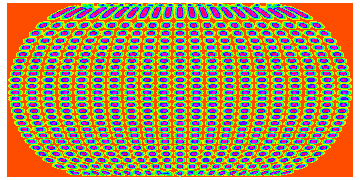Quantum kaleidoscops with BEC in 1D optical lattice with oscillatory interaction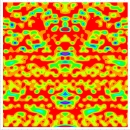Ergodicity of quantum phase in three state model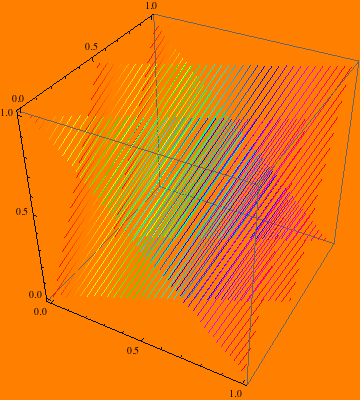Trojan wave packets (or Bohr atom if someone cares less about quantization - Rutherford-Nagaoka atom) observed in the lab:

Arbitrary multipulse fieldcan generate arbitrary quantum state from other arbitrary state within the Hydrogen all bound states manifold but can one generate high angular momentum Trojan Wavepacket directly with one "pulse" from easy to prepare zero angular momentum static field Stark State ?:

I originally discovered Trojan wave packets as follows: The Hamiltonian in two dimensions in circular coordinates is: H=-hbar^2/2m(d^2/r^2 dphi^2 + 1/r d/dr + d^2/dr^2) - w (hbar/i)(d/dphi) - 1/r - e r Cos(phi) . First I assumed that the wave function has a large angular momentum phase simply Psi -> Psi Exp(i l phi). The new wave function Psi fulfills the Schrodinger equation with the Hamiltonian H= -hbar^2/2m d^2/dr^2 +hbar^2/2m (l^2/r^2) - 1/r - hbar^2/2 m r^2 d^2/dphi^2 -i l hbar^2/m r^2 d/ dphi - w (hbar/i)(d/dphi) - e r Cos(phi). This Hamiltonian is still nonseparable so I applied Hartree approximation to the coordinate as it was of 1D particle. Simply H = H_r + H_phi, H_r= -hbar^2/2m d^2/dr^2 +hbar^2/2m (l^2/r^2) - 1/r and H_phi = - hbar^2/2 m r_0^2 d^2/dphi^2 - e r_0 Cos(phi) where selfconsistently Hartree averages were used r_0=Av(r), Av(d/dphi)=0, Av(d/dr)=0 to decouple to separability. The wave function is therefore a product of an approximate Gaussian in r localized around r_0 from locally harmonic normal centrifugal potential + Coulomb potential and the Mathieu function in phi. Trojan wave packet is here the localized inverted pendulum state and the anty-Trojan the ground state. This is opposite to the more exact Mathieu theory, the spectrum is inverted and of the pendulum mass 1 but not -1/3 and the exact classical stability of equilibrium points is opposite but it predicts both packets right where they are at phi=0 and phi=Pi points having theangular momentum hbar l= m w r_0^2/hbar and approximately right energies. The natural consequence is the existence of double-Trojan states in Helium and the mutual stabilization of two parallel Trojan trajectories in hoop earrings configuration in the magnetic field:Trojan wave packets of Deuterium nuclei and their fusion products in Palladium, Helium and Tritium may be an ultimate solution of cold fussion theory as simply normal hot fussion when the Deuterium nucleus in Trojan state supported by the time-dependent fields of passing charges of Palladium lattice makes a critical collision path slaloming on trajectories that are Trojan-like in pieces in small critical bulk mass to cause the fusion-collision-fusion chain reaction in closely packed Deuterium because of the dense space localization of curly trajectory (normally of the order of kiloton in Platinium Deuteride). Because the primary chain reaction would be fusion - Rutherford scattering of products 3T1+ and p+ with Deuterium + (activation) - 2 x fusion and the Deuterium nuclei are very small to heavy elements the critical radius of Palladium sphere to start the cascade without slaloming is much above the fission devices and above 10 meters and the critical mass of Deuterium saturated Palladium is above 10 000 tons. It is essential to improve the trajectories with cyclotronic slaloming with the alternating ultra-strong magnetic field or otherwise to reduce the bulk.